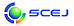## \$B9V1i%W%m%0%i%`!J2q>l!&F|DxJL!K(B

English page

### E \$B2q>l!&Bh(B 2 \$BF|(B

\$B:G=*99?7F|;~!'(B2019-12-10 12:54:57
\$B9V1i(B
\$B;~9o(B
\$B9V1i(B
\$BHV9f(B
\$B9V1iBjL\!?H/I=\$B%-!<%o!<%I(B\$BJ,N`(B
\$BHV9f(B
\$B\$BHV9f(B
\$B%(%M%k%.!<(B, \$B%(%l%/%H%m%K%/%9(B, \$B4D6-(B, \$B9-NN0h(B
(10:00\$B!A(B12:20) (\$B:BD9(B \$BA0ED(B \$B8w<#!&?7A%(B \$B9,Fs(B)
10:00\$B!A(B 10:20E204\$BN3;R(B-\$BN3;R4V\$K\$*\$1\$kEAG.Dq93\$N?tCM2r@O(B
(\$B2,;3Bg1!<+(B) (\$B3X(B)\$B!{@&K\(B \$B>-8c(B\$B!&(B (\$B@5(B)\$BCfA>(B \$B9@0l(B\$B!&(B (\$B@5(B)\$B;0Ln(B \$BBY;V(B\$B!&(B (\$B@5(B)\$B8eF#(B \$BK.>4(B
Thermal resistance
Particle
Packed bed
9-b126
10:20\$B!A(B 10:40E205\$B8GBN9bJ,;R7AG3NAEECS\$N;@AG4T85H?1~B.EY%Q%i%a!<%?!<\$NB,DjK!\$N3+H/(B
(\$B5~Bg9)(B) (\$B3X(B)\$B!{>.@n(B \$B51(B\$B!&(B (\$B3X(B)\$BB<@%(B \$B3pB?(B\$B!&(B (\$B@5(B)\$B;38}(B \$BOB9((B\$B!&(B (\$B@5(B)\$B1F;3(B \$BH~HA(B\$B!&(B (\$B@5(B)\$B2O@%(B \$B85L@(B
polymer electrolyte fuel cell
oxygen reduction reaction
platinum-sputtered-electrode
9-e62
10:40\$B!A(B 11:00E206\$B>o29\$G\$N6u5\$Cf\$+\$i\$NEE5\$2=3XE*;@AGJ,N%\$K4X\$9\$k4pAC;n83(B
(\$BBg9)Bg(B) (\$B3X(B)\$B!{0a3^(B \$BB@0l(B\$B!&(B (\$B3X(B)\$B?eFb(B \$BCR5.(B\$B!&(B (\$B@5(B)\$B5\K\(B \$B6Q(B
Oxygen separation
Ion exchange membrane
Electrochemistry
11-a23
11:00\$B!A(B 11:20E207\$BEE5\$2=3XH?1~\$K\$h\$k?eAG\$H%"%;%H%"%k%G%R%I\$NF1;~@=B\$%W%m%;%9\$N3+H/(B
(\$B5~Bg9)(B) (\$B3X(B)\$B!{L>B<(B \$B7\$B!&(B (\$B3X(B)\$BLJLn(B \$BL4Bg(B\$B!&(B (\$B@5(B)\$B2O@%(B \$B85L@(B
electroorganic synthesis
potential difference
11-a98
11:20\$B!A(B 11:40E208\$B%W%i%9%A%C%/\$K5[Ce\$7\$?M-5!1x@w2=9gJ*\$N8wJ,2r5sF0\$NI>2A(B
(\$B9-Bg1!9)(B) (\$B3X(B)\$B!{3k>e(B \$BFX;K(B\$B!&(B (\$B@5(B)\$B@>Eh(B \$B>D(B\$B!&(B (\$B@5(B)\$BCf0f(B \$BCR;J(B\$B!&(B (\$B@5(B)\$B8eF#(B \$B7rI'(B\$B!&(B Nazmul Hassan
organic pollutants
plastics
13-f66
11:40\$B!A(B 12:00E209\$B6bB02C9)%W%m%;%9\$K\$*\$\$\$FH/@8\$9\$k%*%\$%k%_%9%H\$N7WB,\$HJa=8(B
(\$B6bBtBg<+A32J(B) (\$B3X(B)\$B!{;{Eh(B \$B9I51(B\$B!&(B \$BJ?0f(B \$B9,\$B!&(B (\$B;05!9)6H(B) (\$B3X(B)\$B4d:j(B \$B\$B!&(B \$BJ!?9(B \$B44B@(B\$B!&(B (\$B6bBtBgM}9)(B) \$BCv8T(B \$BLo@8(B\$B!&(B (\$B@5(B)\$BEl(B \$B=(7{(B\$B!&(B (\$B@5(B)\$B@%8M(B \$B>OJ8(B
oil mist
nanoparticle
13-i140
12:00\$B!A(B 12:20E210\$BM}7O3X@8\$N\$?\$a\$N%0%m!<%P%k2J3X650i(B
(\$BJ<8K8)BgAm9g(B) (\$B@5(B)\$BBgFb(B \$B44M:(B
Global Science
English for Engineers
Sustainable Development
14-c130

\$B9V1iH/I=%W%m%0%i%`(B
\$B2=3X9)3X2q(B \$BI1O)Bg2q(B2019(C) 2019 \$B8x1W
Most recent update: 2019-12-10 12:54:57
E-mail: inquiry-ks2019www3.scej.org
This page was generated byeasp 2.44; proghtml 2.42 (C)1999-2018 kawase